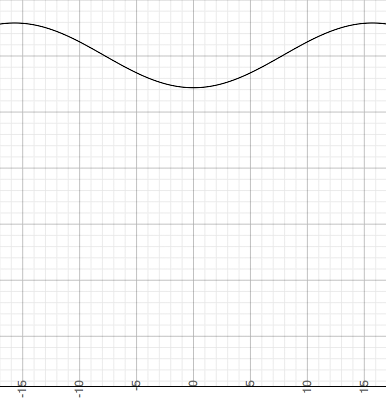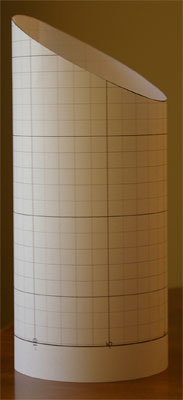SEARCH HOMEMath Central Quandaries & QueriesQuestion from John: from the original question Al asked about cutting a 200 diameter pipe in 45 degrees. can someone explain the math steps required to creating the graph. I am trying to do the same thing only using a 150 degree cut. Thanks, JohnHi John,

Most of the information you need is in Edwards response to Neal's question and my response to Al's question.

Suppose the diameter of the pipe is d units and the pipe is sliced by a plane in inclination φ degrees, in your case 150 degrees. Following Edward's development the equation of the intersecting plane is z = tan(φ) x which in your example is z = tan(150o) x = -1/√3 x. The equation of the curve you need is then

y = d/2 tan(φ) cos(2x/d)

where the angle 2x/d is in radians. In your case where the plane that slices the pipe has an inclination of 150o this is

y = -d/(2√3) cos(2x/d)

For example I took d = 10 units, graphed one period of y = -5/√3) cos(x/5)cut it out and rolled it up to form the pipeHarleyMath Central is supported by the University of Regina and The Pacific Institute for the Mathematical Sciences.